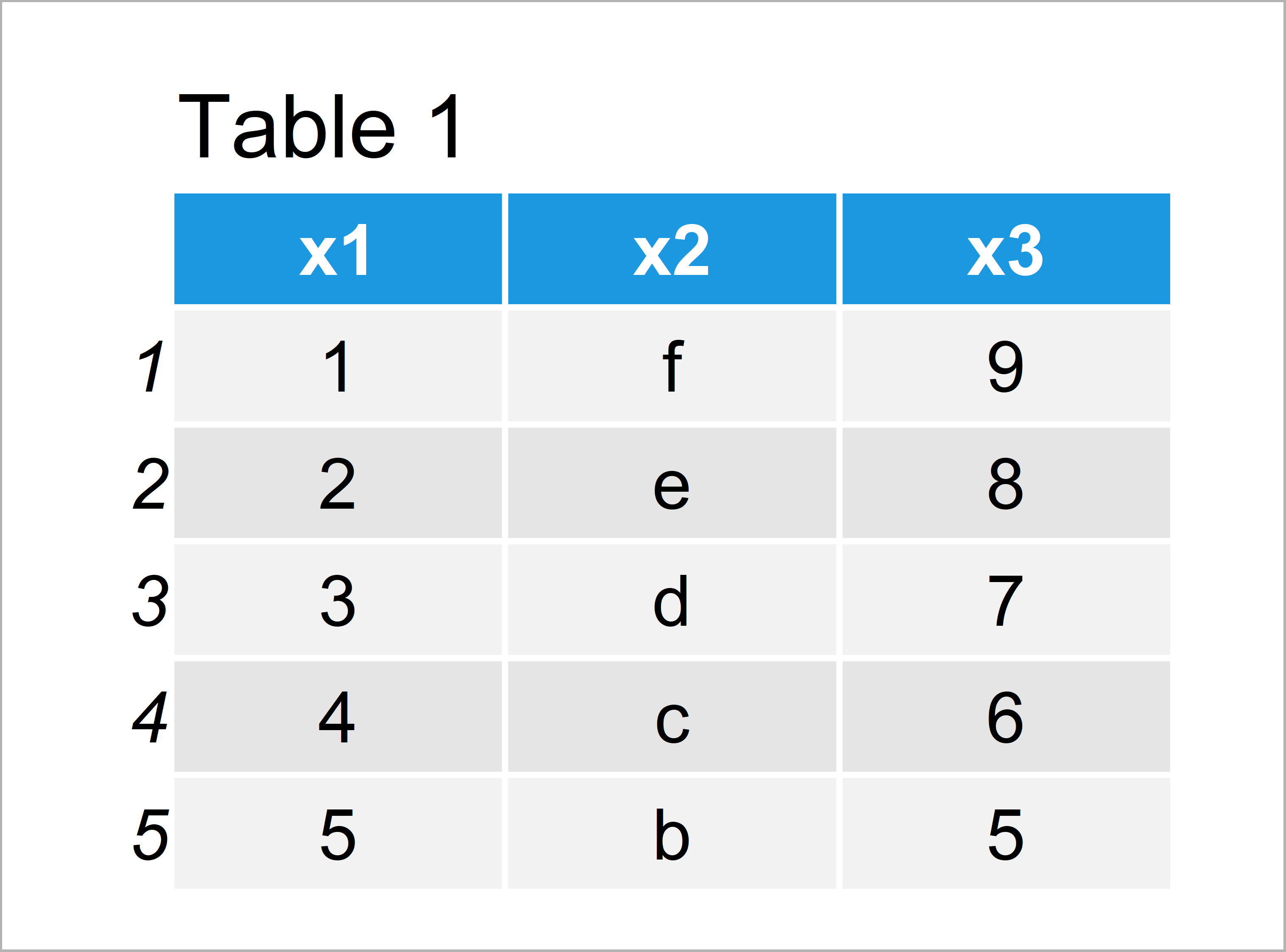# Convert Column Classes of Data Table in R (2 Examples)

In this R tutorial you’ll learn how to convert the data types of the variables of a data.table.

The tutorial will contain two examples for the conversion of data classes. To be more precise, the article contains this information:

Sound good? Let’s dive into it.

## Example Data & Add-On Packages

First, we need to install and load the data.table package:

```install.packages("data.table")                  # Install data.table package

We’ll use the following data table as basement for this R programming tutorial:

```data <- data.table(x1 = 1:5,                    # Create data table
x2 = letters[6:2],
x3 = 9:5)
data                                            # Print data table```As you can see based on Table 1, our example data is a data table consisting of five rows and three columns.

Let’s check the data types of our data table column using the sapply and class functions:

```sapply(data, class)                             # Check classes of data table columns
#          x1          x2          x3
#   "integer" "character"   "integer"```

The RStudio console shows the classes of each of our data table columns: x1 is an integer, x2 is a character, and x3 is also an integer.

## Example 1: Convert Type of One Specific Data Table Variable

The following code shows how to modify the class of only one data table variable.

Have a look at the R syntax below:

`data_new1 <- data[ , x1 := as.character(x1)]    # Convert one column`

The previous R code changed the class of the variable x1 from integer to character

Let’s check the classes of all columns once again:

```sapply(data_new1, class)                        # Check classes of data table columns
#          x1          x2          x3
# "character" "character"   "integer"```

As you can see, only the first variable class was changed.

Note that we could apply the same type of code to convert our data table variables to numeric or factor. We simply would have to use the as.numeric or as.factor functions instead of the as.character function.

## Example 2: Convert Type of Multiple Data Table Variables

In Example 2, I’ll illustrate how to adjust the data type of multiple data table columns.

First, we have to create a vector containing the column names of all variables that we want to convert:

`change_columns <- c("x1", "x3")                 # Specify columns to change`

Next, we can execute the R code below to change the class of all previously specified variables:

```data_new2 <- data                               # Duplicate data table
data_new2[ ,                                    # Change class of certain columns
(change_columns) := lapply(.SD, as.character),
.SDcols = change_columns]```

Let’s check the updated classes:

```sapply(data_new2, class)                        # Check classes of data table columns
#          x1          x2          x3
# "character" "character" "character"```

The classes of the variables x1 and x3 were changed to character.

## Video, Further Resources & Summary

If you need further information on the R syntax of this article, I recommend having a look at the following video of my YouTube channel. In the video, I’m showing the R code of this tutorial in RStudio.

In addition, you might have a look at the related articles of my website. A selection of articles is listed below.

To summarize: In this R programming tutorial you have learned how to change column classes of data.table variables to numeric, character, or factor. Let me know in the comments section, if you have additional questions.

Subscribe to the Statistics Globe Newsletter